Ex 3.3

Chapter 3 Class 6 Playing with Numbers
Serial order wise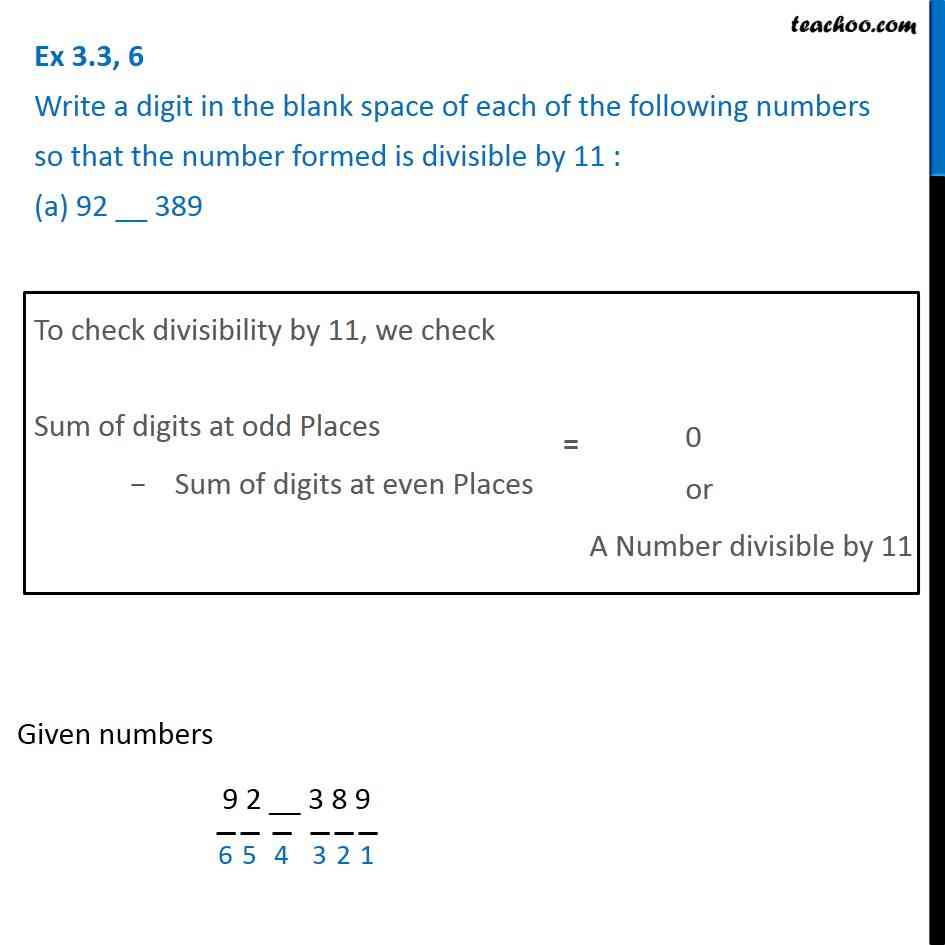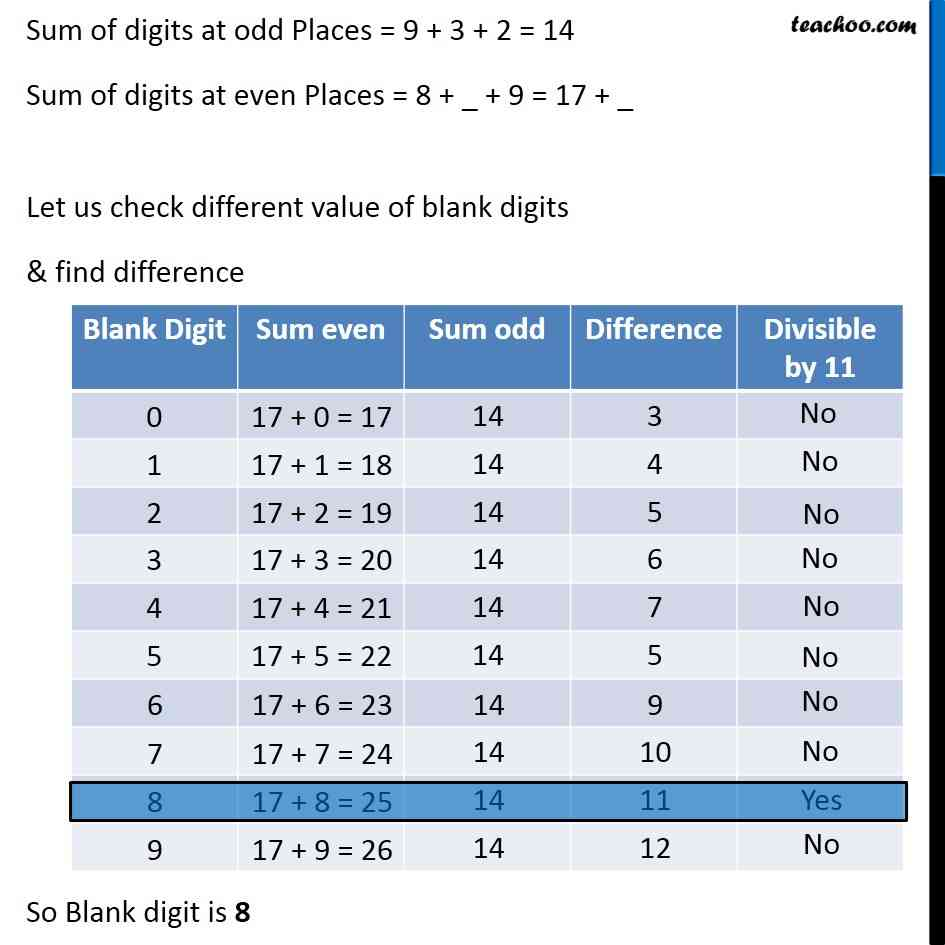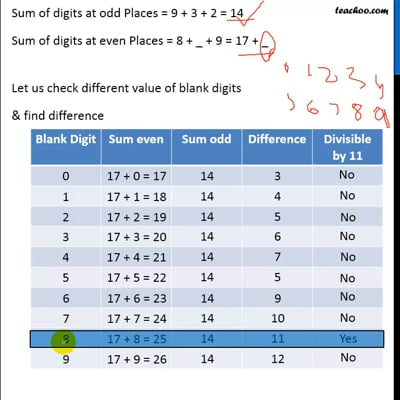This video is only available for Teachoo black users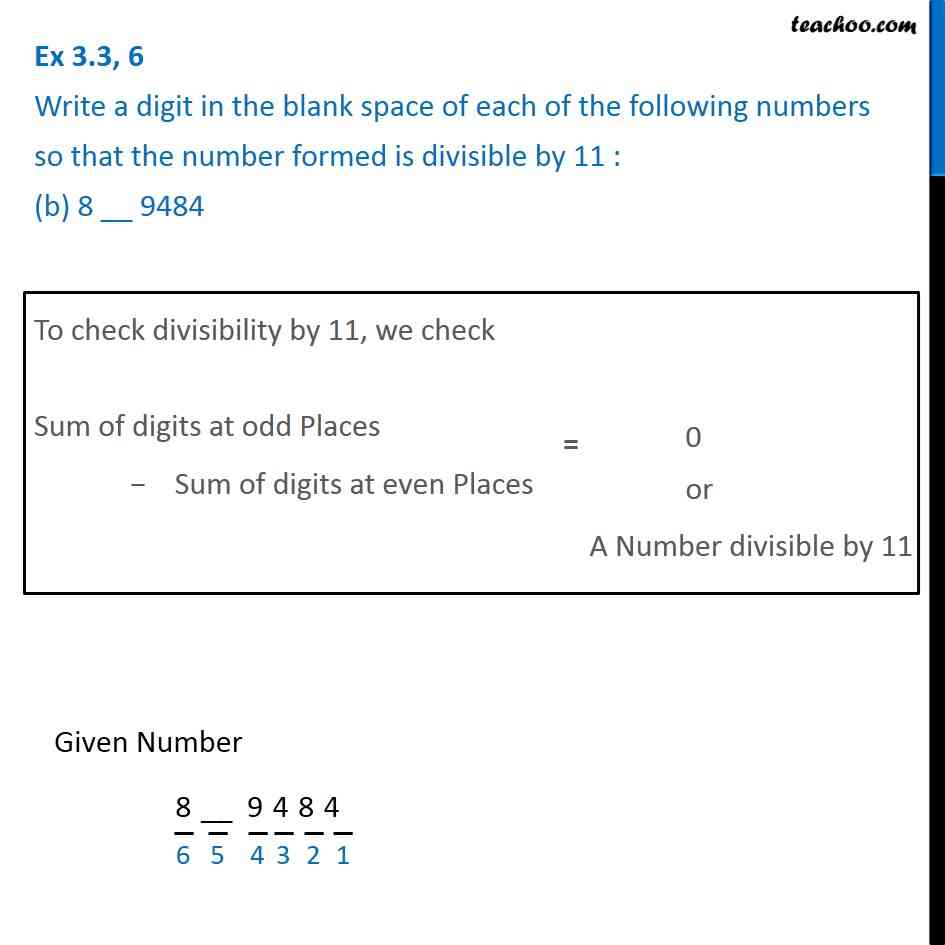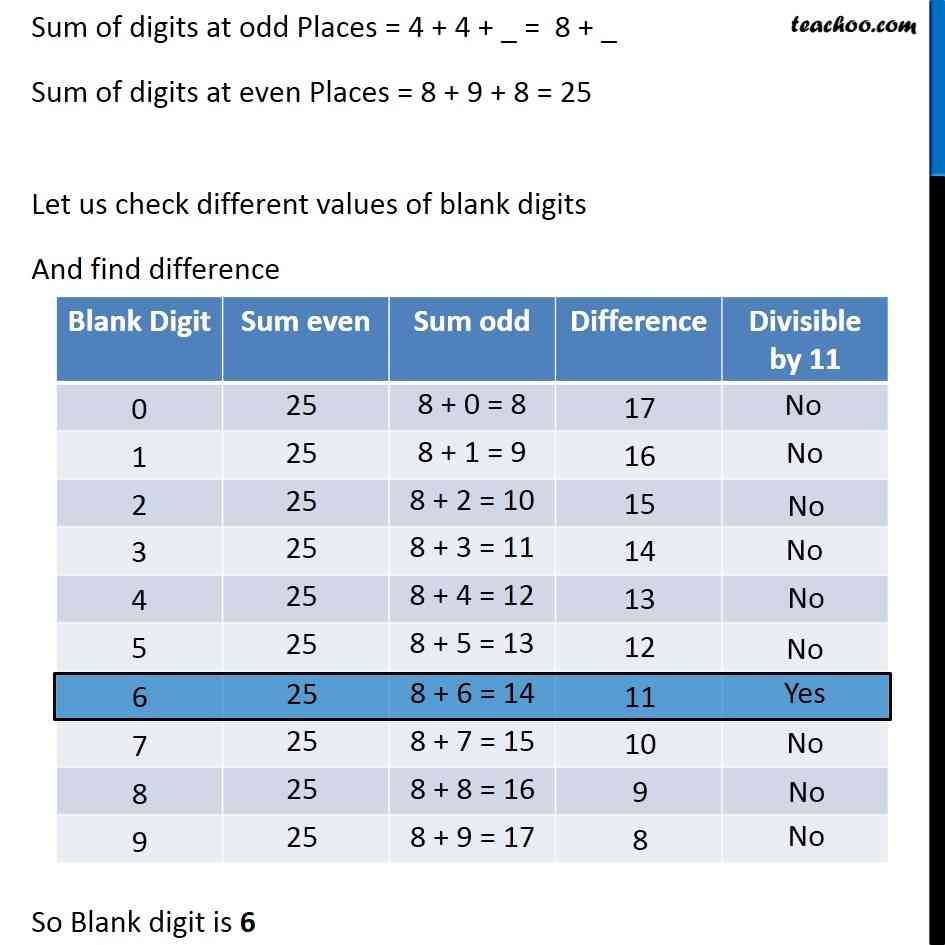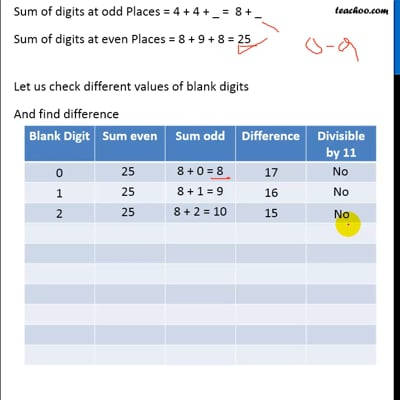This video is only available for Teachoo black users

### Transcript

Ex 3.3, 6 Write a digit in the blank space of each of the following numbers so that the number formed is divisible by 11 : (a) 92 __ 389To check divisibility by 11, we check Sum of digits at odd Places − Sum of digits at even Places Given numbers 9 2 __ 3 8 9 Sum of digits at odd Places = 9 + 3 + 2 = 14 Sum of digits at even Places = 8 + _ + 9 = 17 + _ Let us check different value of blank digits & find difference So Blank digit is 8 Ex 3.3, 6 Write a digit in the blank space of each of the following numbers so that the number formed is divisible by 11 : (b) 8 __ 9484To check divisibility by 11, we check Sum of digits at odd Places − Sum of digits at even Places Given Number 8__ 9484 Sum of digits at odd Places = 4 + 4 + _ = 8 + _ Sum of digits at even Places = 8 + 9 + 8 = 25 Let us check different values of blank digits And find difference So Blank digit is 6